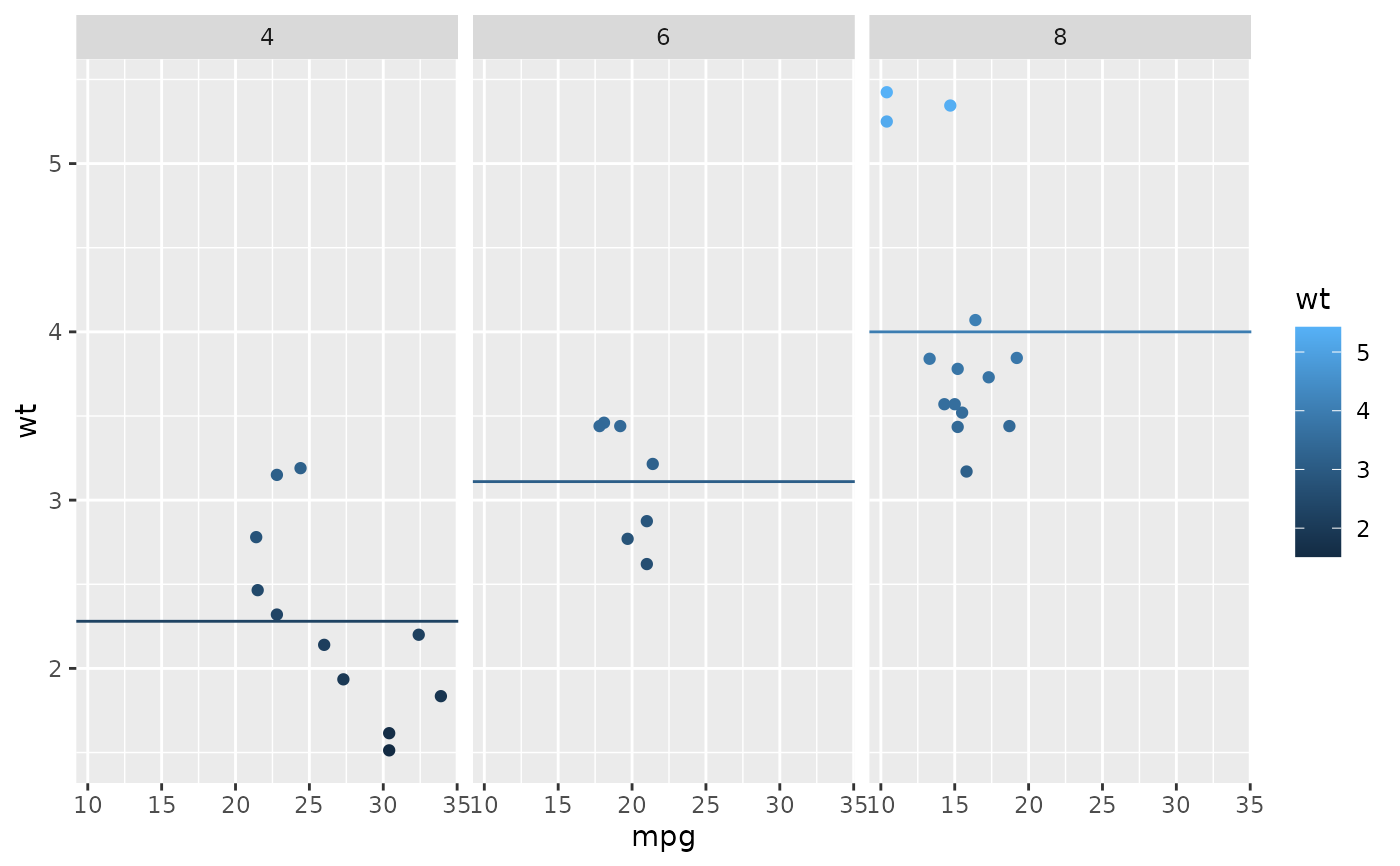These geoms add reference lines (sometimes called rules) to a plot, either horizontal, vertical, or diagonal (specified by slope and intercept). These are useful for annotating plots.

## Usage

geom_abline(
mapping = NULL,
data = NULL,
...,
slope,
intercept,
na.rm = FALSE,
show.legend = NA
)

geom_hline(
mapping = NULL,
data = NULL,
...,
yintercept,
na.rm = FALSE,
show.legend = NA
)

geom_vline(
mapping = NULL,
data = NULL,
...,
xintercept,
na.rm = FALSE,
show.legend = NA
)

## Arguments

mapping

Set of aesthetic mappings created by aes().

data

The data to be displayed in this layer. There are three options:

If NULL, the default, the data is inherited from the plot data as specified in the call to ggplot().

A data.frame, or other object, will override the plot data. All objects will be fortified to produce a data frame. See fortify() for which variables will be created.

A function will be called with a single argument, the plot data. The return value must be a data.frame, and will be used as the layer data. A function can be created from a formula (e.g. ~ head(.x, 10)).

...

Other arguments passed on to layer(). These are often aesthetics, used to set an aesthetic to a fixed value, like colour = "red" or size = 3. They may also be parameters to the paired geom/stat.

na.rm

If FALSE, the default, missing values are removed with a warning. If TRUE, missing values are silently removed.

show.legend

logical. Should this layer be included in the legends? NA, the default, includes if any aesthetics are mapped. FALSE never includes, and TRUE always includes. It can also be a named logical vector to finely select the aesthetics to display.

xintercept, yintercept, slope, intercept

Parameters that control the position of the line. If these are set, data, mapping and show.legend are overridden.

## Details

These geoms act slightly differently from other geoms. You can supply the parameters in two ways: either as arguments to the layer function, or via aesthetics. If you use arguments, e.g. geom_abline(intercept = 0, slope = 1), then behind the scenes the geom makes a new data frame containing just the data you've supplied. That means that the lines will be the same in all facets; if you want them to vary across facets, construct the data frame yourself and use aesthetics.

Unlike most other geoms, these geoms do not inherit aesthetics from the plot default, because they do not understand x and y aesthetics which are commonly set in the plot. They also do not affect the x and y scales.

## Aesthetics

These geoms are drawn using geom_line() so they support the same aesthetics: alpha, colour, linetype and linewidth. They also each have aesthetics that control the position of the line:

• geom_vline(): xintercept

• geom_hline(): yintercept

• geom_abline(): slope and intercept

See geom_segment() for a more general approach to adding straight line segments to a plot.

## Examples

p <- ggplot(mtcars, aes(wt, mpg)) + geom_point()

# Fixed values
p + geom_vline(xintercept = 5)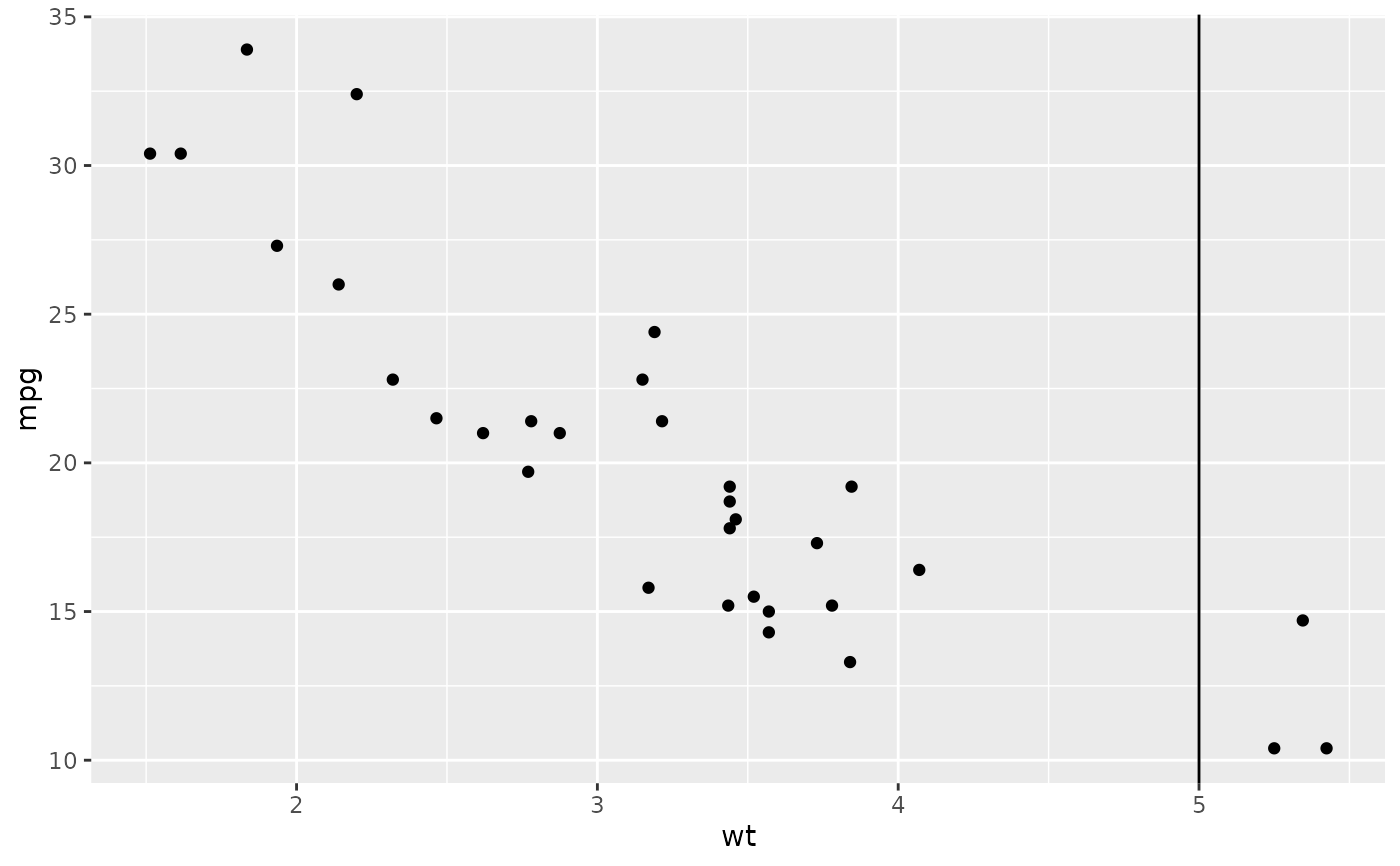p + geom_vline(xintercept = 1:5)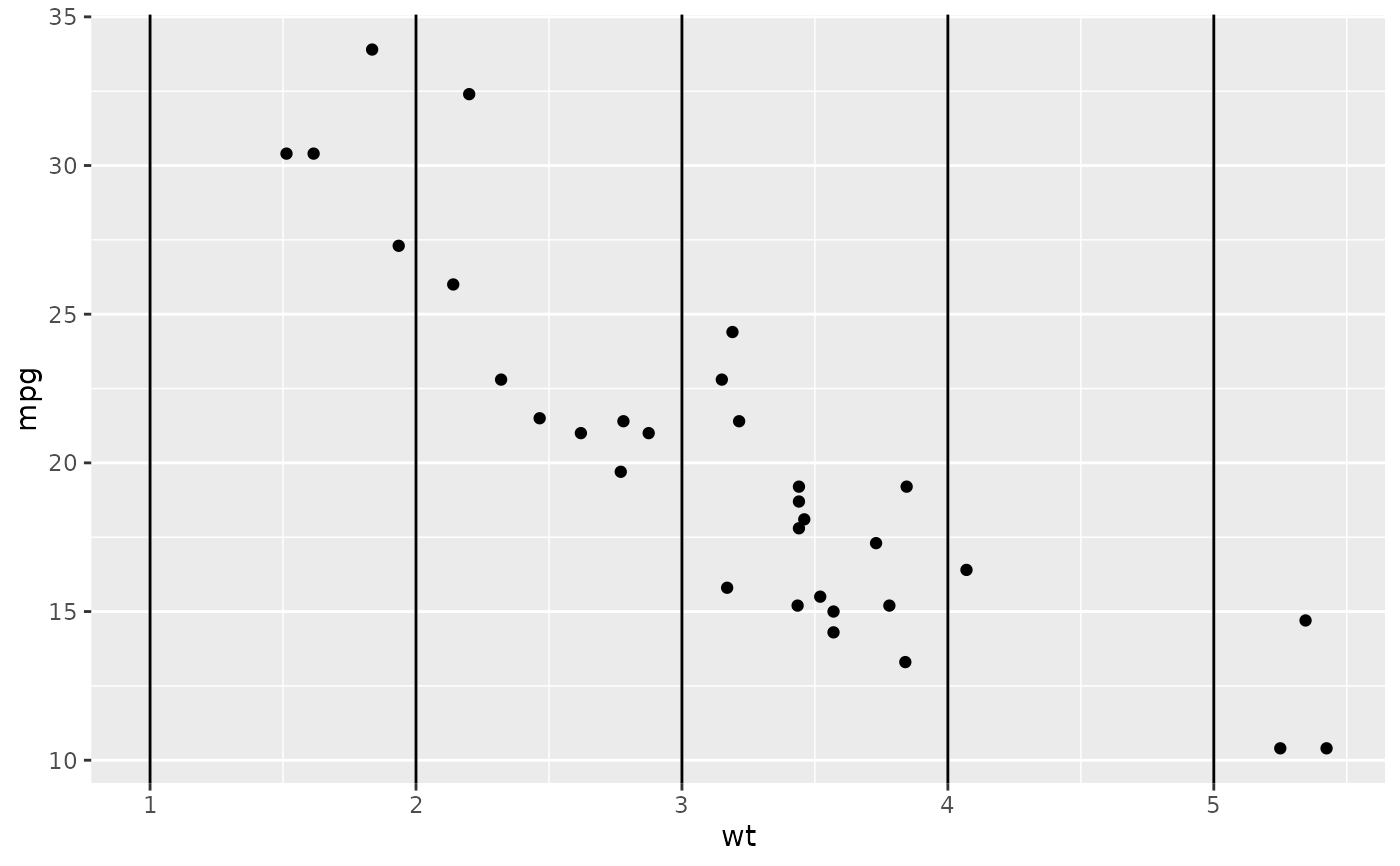p + geom_hline(yintercept = 20)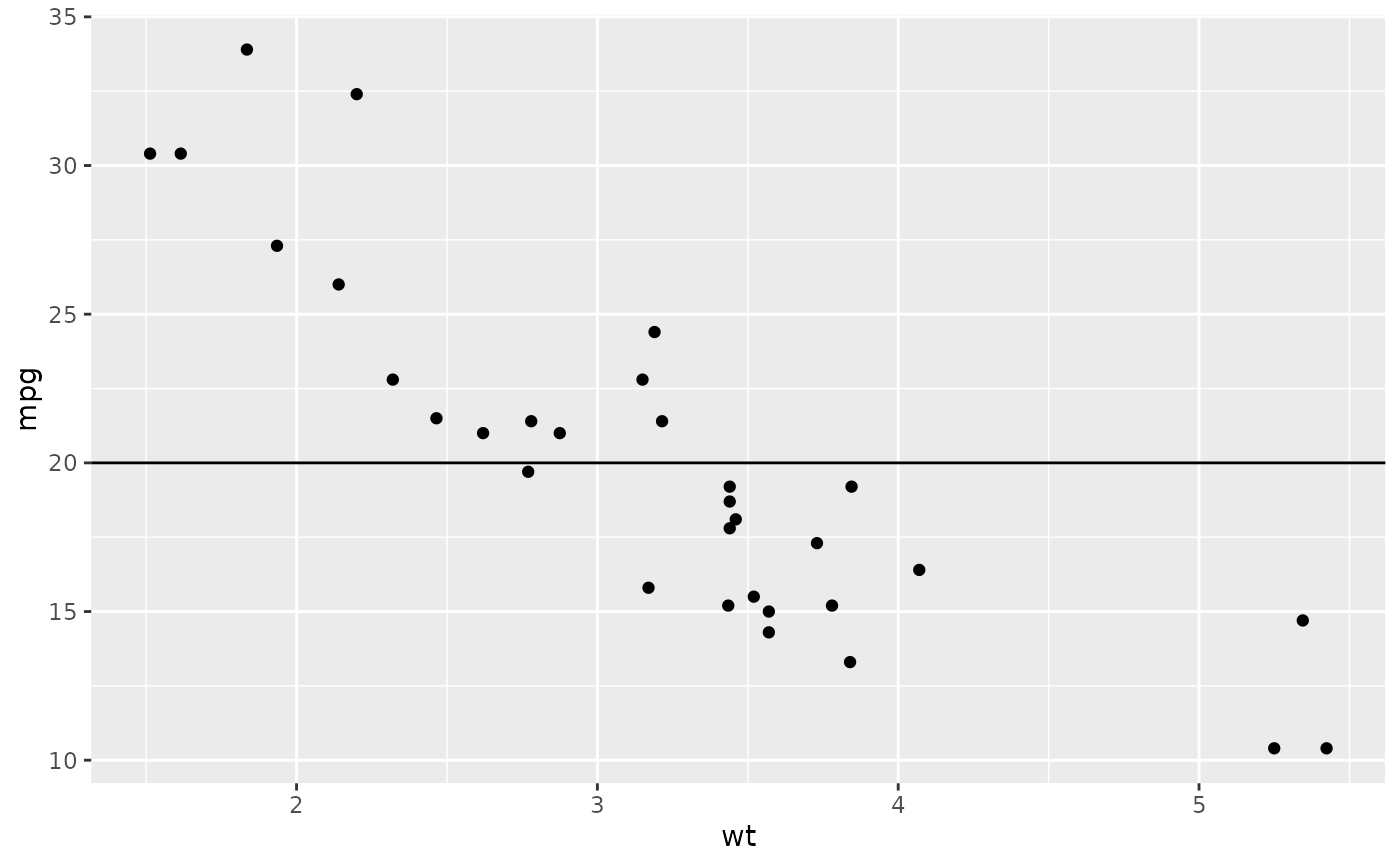p + geom_abline() # Can't see it - outside the range of the data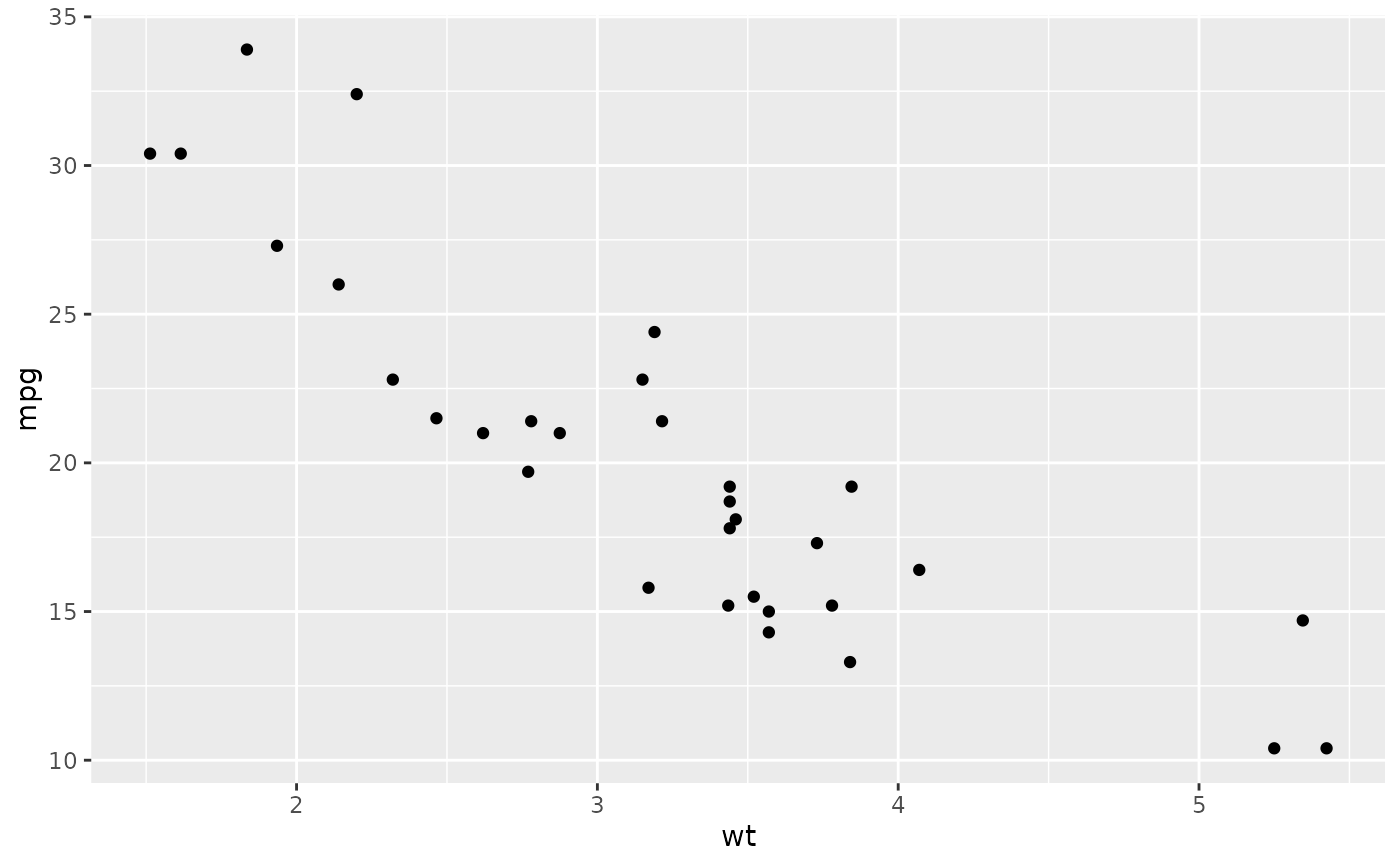p + geom_abline(intercept = 20)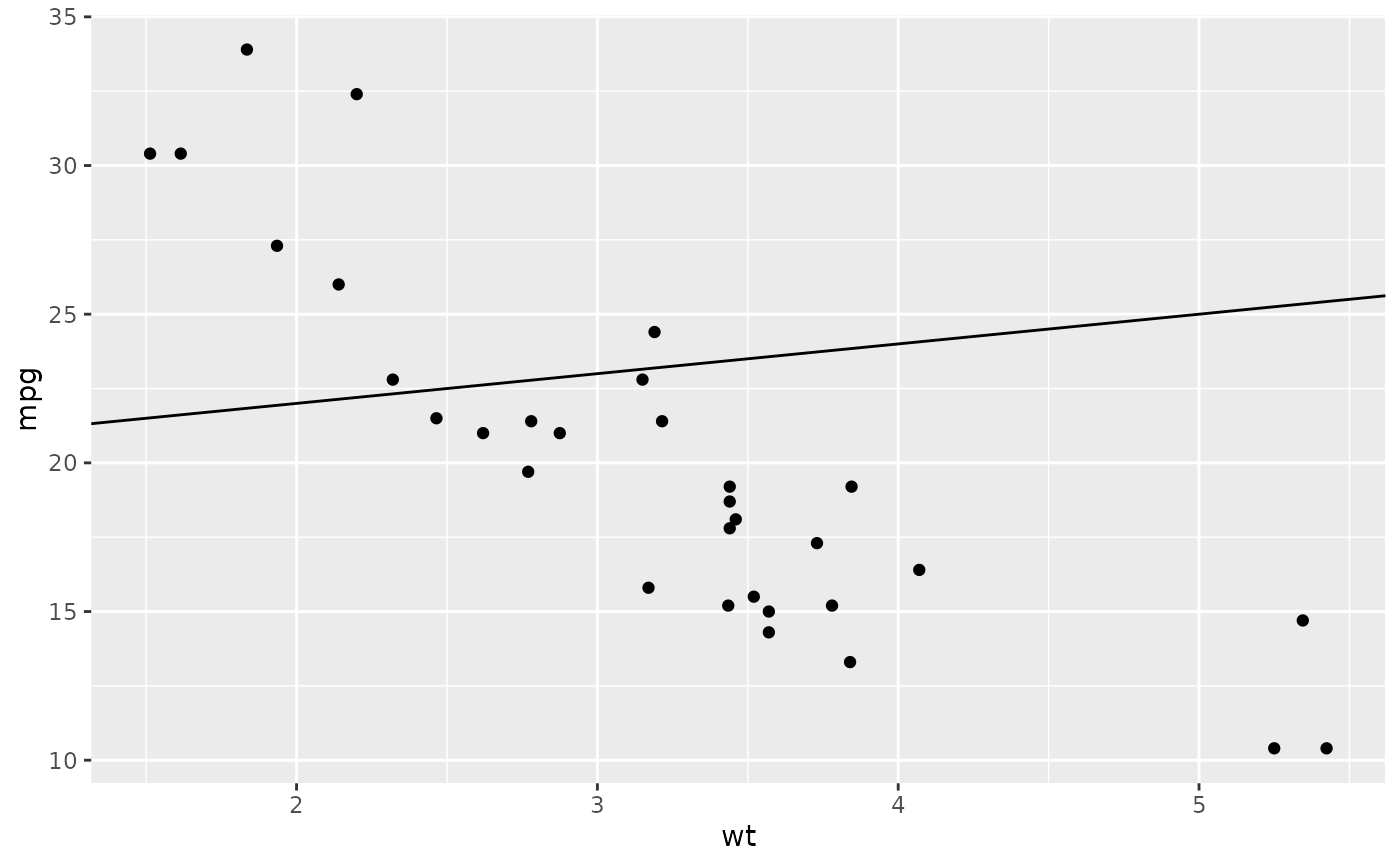# Calculate slope and intercept of line of best fit
coef(lm(mpg ~ wt, data = mtcars))
#> (Intercept)          wt
#>   37.285126   -5.344472
p + geom_abline(intercept = 37, slope = -5)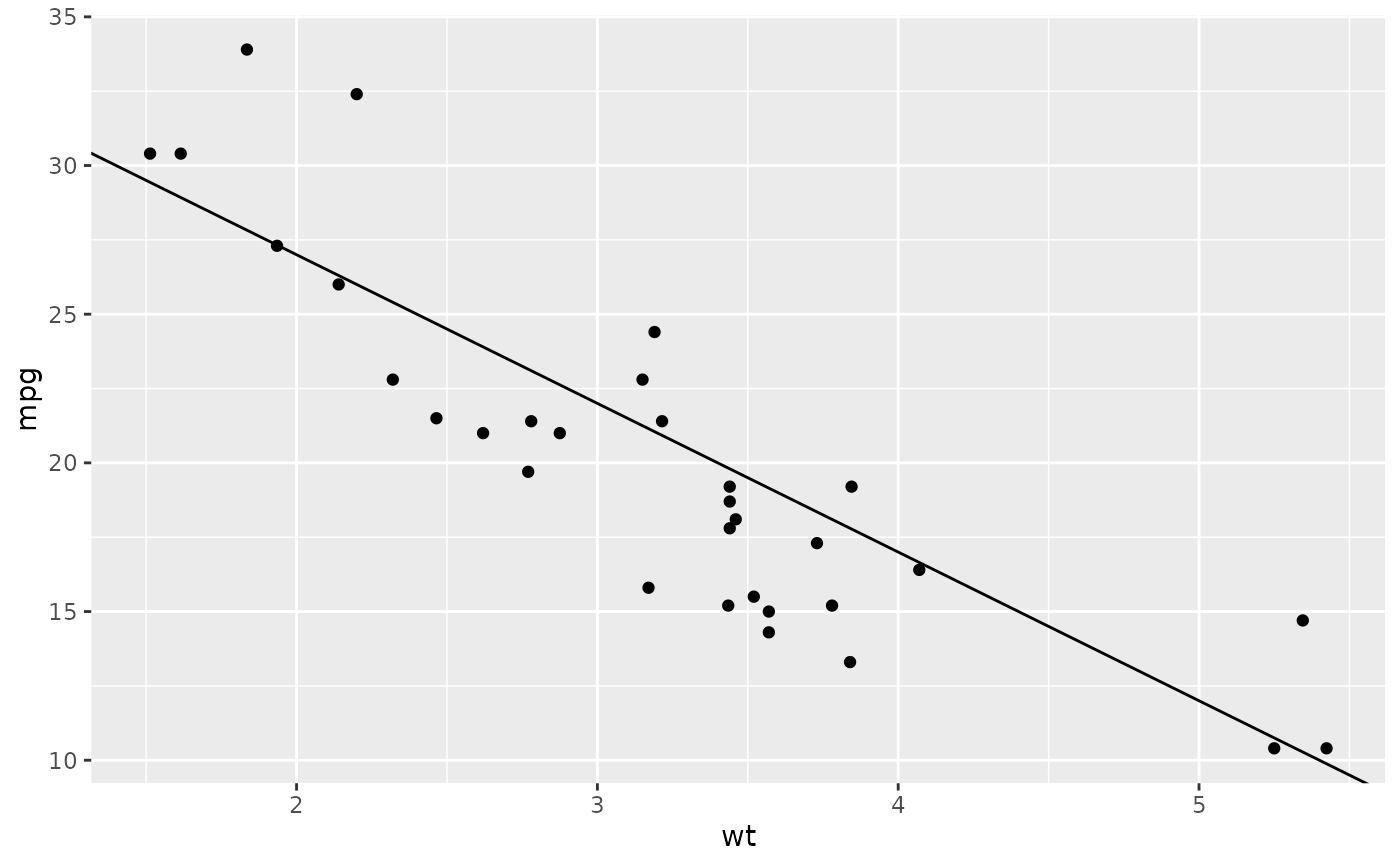# But this is easier to do with geom_smooth:
p + geom_smooth(method = "lm", se = FALSE)
#> geom_smooth() using formula = 'y ~ x'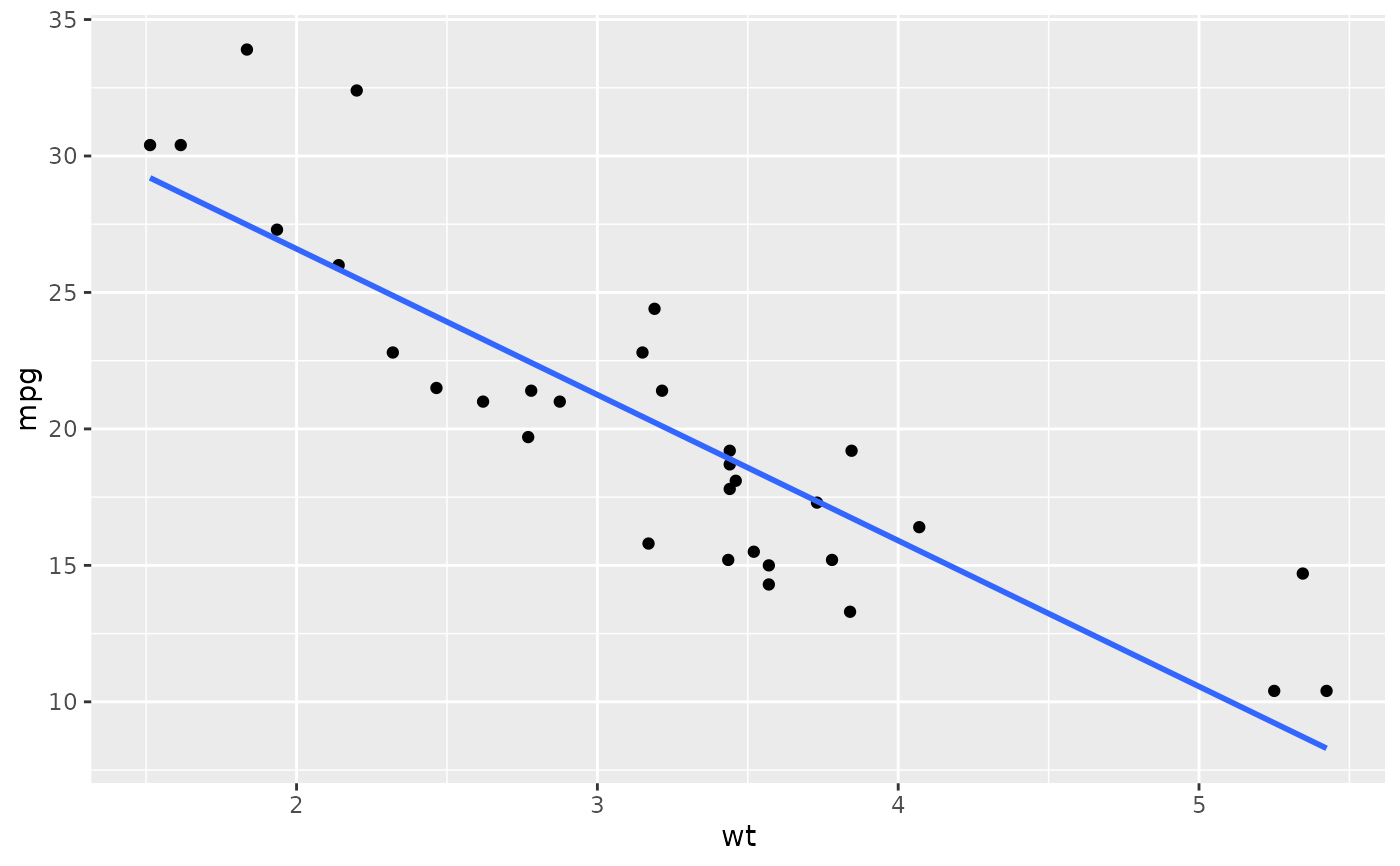# To show different lines in different facets, use aesthetics
p <- ggplot(mtcars, aes(mpg, wt)) +
geom_point() +
facet_wrap(~ cyl)

mean_wt <- data.frame(cyl = c(4, 6, 8), wt = c(2.28, 3.11, 4.00))
p + geom_hline(aes(yintercept = wt), mean_wt)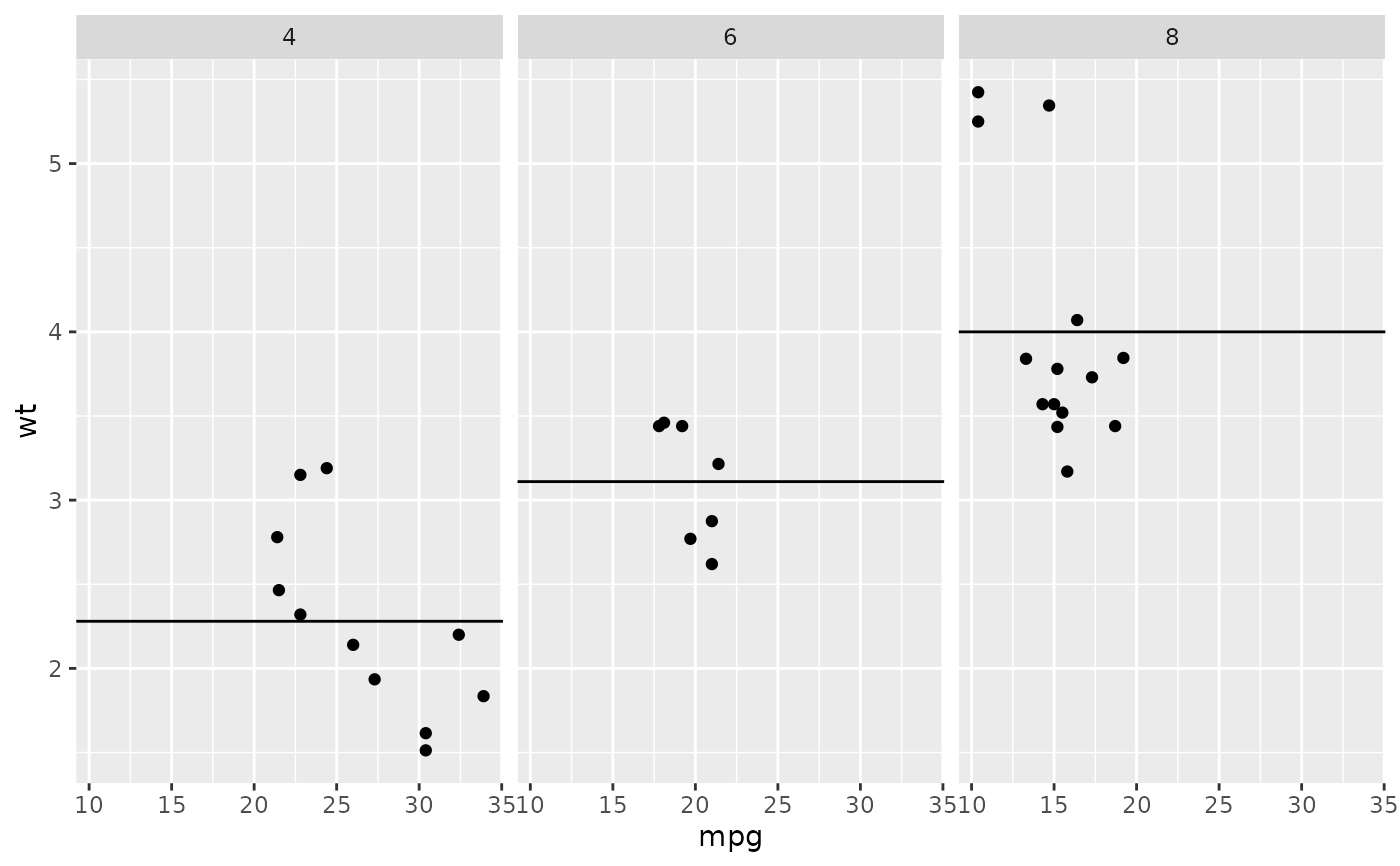# You can also control other aesthetics
ggplot(mtcars, aes(mpg, wt, colour = wt)) +
geom_point() +
geom_hline(aes(yintercept = wt, colour = wt), mean_wt) +
facet_wrap(~ cyl)